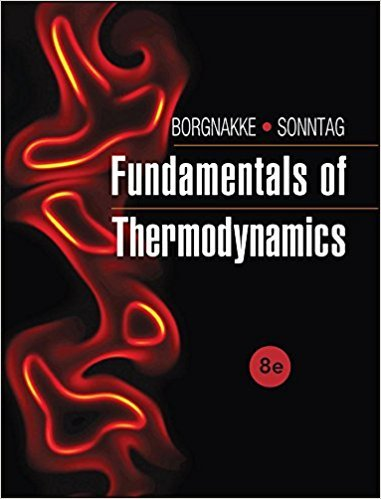×
Get Full Access to Fundamentals Of Thermodynamcs - 8 Edition - Chapter 1 - Problem 71hp
Get Full Access to Fundamentals Of Thermodynamcs - 8 Edition - Chapter 1 - Problem 71hp

×

# The pressure gauge on an air tank shows 75 kPa when theISBN: 9781118131992 58

## Solution for problem 71HP Chapter 1

Fundamentals of Thermodynamcs | 8th Edition

• Textbook Solutions
• 2901 Step-by-step solutions solved by professors and subject experts
• Get 24/7 help from StudySoup virtual teaching assistantsFundamentals of Thermodynamcs | 8th Edition

4 5 1 388 Reviews
18
5
Problem 71HP

Problem 71HP

The pressure gauge on an air tank shows 75 kPa when the diver is 10 m down in the ocean. At what depth will the gauge pressure be zero? What does that mean?

Step-by-Step Solution:

Solution 71HP

Step 1 of 2

We have to find at what depth will be the gauge pressure equal to zero and what does it mean, if the pressure gauge on an air tank shows 75 kPa when the diver is 10 m down in the ocean.

The hydrostatic pressure at the depth of 10 m is given by the expression,Where,atmospheric pressure

= 101,325 Pakg/m3m/s2m

Thus,Step 2 of 2

##### ISBN: 9781118131992

Since the solution to 71HP from 1 chapter was answered, more than 500 students have viewed the full step-by-step answer. The answer to “The pressure gauge on an air tank shows 75 kPa when the diver is 10 m down in the ocean. At what depth will the gauge pressure be zero? What does that mean?” is broken down into a number of easy to follow steps, and 33 words. This full solution covers the following key subjects: Gauge, pressure, mean, down, air. This expansive textbook survival guide covers 7 chapters, and 1462 solutions. The full step-by-step solution to problem: 71HP from chapter: 1 was answered by , our top Engineering and Tech solution expert on 08/03/17, 05:05AM. This textbook survival guide was created for the textbook: Fundamentals of Thermodynamcs , edition: 8. Fundamentals of Thermodynamcs was written by and is associated to the ISBN: 9781118131992.

Unlock Textbook Solution